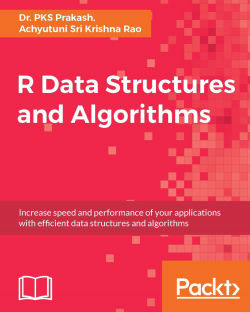# R Data Structures and Algorithms

By Dr. PKS Prakash , Achyutuni Sri Krishna Rao

• Constantly updated with 100+ new titles each month
• Breadth and depth in over 1,000+ technologies

In this book, we cover not only classical data structures, but also functional data structures.

We begin by answering the fundamental question: why data structures? We then move on to cover the relationship between data structures and algorithms, followed by an analysis and evaluation of algorithms. We introduce the fundamentals of data structures, such as lists, stacks, queues, and dictionaries, using real-world examples. We also cover topics such as indexing, sorting, and searching in depth.

Later on, you will be exposed to advanced topics such as graph data structures, dynamic programming, and randomized algorithms. You will come to appreciate the intricacies of high performance and scalable programming using R. We also cover special R data structures such as vectors, data frames, and atomic vectors.

With this easy-to-read book, you will be able to understand the power of linked lists, double linked lists, and circular linked lists. We will also explore the application of binary search and will go in depth into sorting algorithms such as bubble sort, selection sort, insertion sort, and merge sort.

Publication date:
November 2016
Publisher
Packt
Pages
276
ISBN
9781786465153

## Chapter 1. Getting Started

Faster and efficient information retrieval is the primary objective of most computer programs. Data structures and algorithms help us in achieving the objective by processing and retrieving data faster. Information retrieval can easily be integrated with algorithms to answer inferential questions from data such as:

• How are sales increasing over time?

• What is customer's arrival distribution over time?

• Out of all the customers who visit between 3:00 and 6:00 PM, how many order Asian versus Chinese?

• Of all the customers visiting, how many are from the same city?

In the efficient processing of the preceding queries, especially in big data scenarios, data structures and algorithms utilized for data retrieval play a significant role. This book will introduce primary data structures such as lists, queues, and stacks, which are used for information retrieval and trade-off of different data structures. We will also introduce the data structure and algorithms evaluation approach for retrieval and processing of defined data structures.

Algorithms are evaluated based on complexity and efficiency, where complexity refers to an algorithm design which is easy to program and debug, and efficacy ensures that the algorithm is utilizing computer resources optimally. This book will focus on the efficiency part of algorithms using data structures, and the current chapter introduces the importance of data structure and the algorithms used for retrieval of data from data structures.

## Introduction to data structure

Moore's law in 1965 observed that the number of transistors per square inch in a dense integrated circuit (IC) had doubled every year since its invention, thus enhancing computational power per computer. He revised his forecast in 1975, stating that the number of transistors would double every 2 years, instead of every year, due to saturation: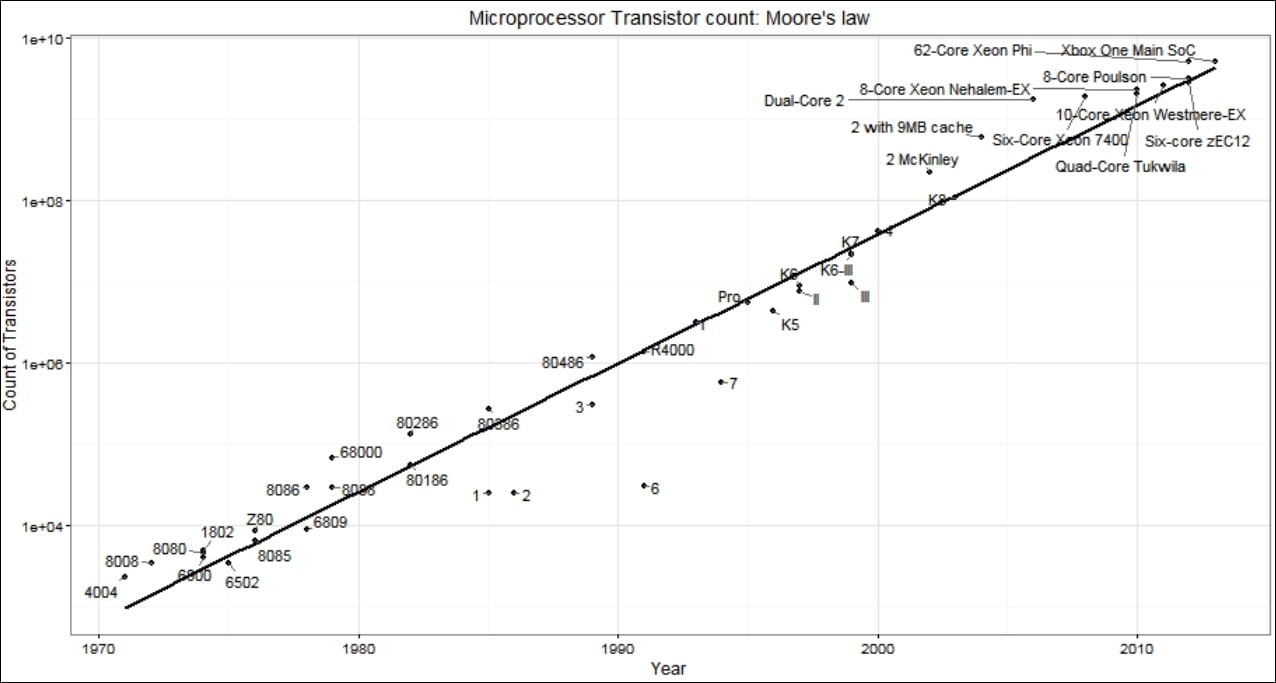Figure 1.1: Moore's law (Ref: data credit - Transistor count, Wikipedia)

Also, although the computational power has been increasing, problem complexity and data sources have also been increasing exponentially over the decade, enforcing the need for efficient algorithms: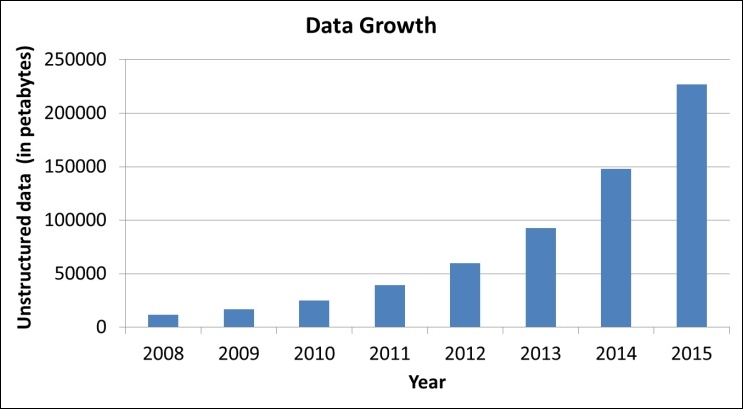Figure 1.2: Increase in size of unstructured data (Ref: Enterprise strategy group 2010)

This explosion in data from 2008 to 2015 has led to a new field of data science where people put in a lot of effort to derive insights using all kinds of datasets such as structured, semi-structured, and unstructured. Thus, to efficiently deal with data scalability, it is important to efficiently store and retrieve datasets. For example, searching for a word in a dictionary would take a long time if the data is randomly organized; thus, sorted list data structures are utilized to ensure a faster search of words. Similarly, searching for an optimal path in a city based on the input location requires data about road network, position, and so on to be stored in the form of geometries. Ideally, even a variable stored as any built-in data type such as character, integer, or float can be considered as a data structure of scalar nature. However, data structure is formally defined as a scheme of organizing related information in a computer so that it can be used efficiently.

Similarly, for algorithms, given sufficient space and time, any dataset can be stored and processed to answer questions of interest. However, selecting the correct data structure will help you save a lot of memory and computational power. For example, assigning a float to integer data type, such as the number of customers coming in a day, will require double the memory. However, in the real-world scenario, computers are constrained by computational resources and space. Thus, a solution can be stated as efficient if it is able to achieve the desired goal in the given resources and time. Thus, this could be used as a cost function to compare the performance of different data structures while designing algorithms. There are two major design constraints to be considered while selecting the data structure:

• Analyze the problem to decide on the basic operation that must be supported into the selected data structure, such as inserting an item, deleting an item, and searching for an item

• Evaluate the resource constraint for each operation

The data structure is selected depending on the problem scenario, for example, a case where complete data is loaded at the beginning, and there is no change/insertion in data, requires similar data structure. However, similar including deletion into data structure will make data structure implementation more complex.

### Tip

Detailed steps to download the code bundle are mentioned in the Preface of this book. Please have a look. The code bundle for the book is also hosted on GitHub at https://github.com/PacktPublishing/R-Data-Structures-and-Algorithms. We also have other code bundles from our rich catalog of books and videos available at https://github.com/PacktPublishing/. Check them out!

## Abstract data type and data structure

The abstract data type (ADT) is used to define high-level features and operations for data structure, and is required before we dig down into details of implementation of data structure. For example, before implementing a linked list, it would be good to know what we want to do with the defined linked list, such as:

• I should be able to insert an item into the linked list

• I should be able to delete an item from the linked list

• I should be able to search an item in the linked list

• I should be able to see whether the linked list is empty or not

The defined ADT will then be utilized to define the implementation strategy. We will discuss ADT for different data structures in more detail later in this book. Before getting into definitions of ADT, it's important to understand data types and their characteristics, as they will be utilized to build an ecosystem for the data structures.

Data type is a way to classify various types of data such as Boolean, integer, float, and string. To efficiently classify a dataset, the following characteristics are needed in any data type:

• Atomic: It should define a single concept

• Traceable: It should be able to map with the same data type

• Accurate: It should be unambiguous

• Clear and concise: It should be understandable

Data types can be classified into two types:

• Built-in data type

• Derived data type

Data types for which a language has built-in support are known as built-in data types. For example, R supports the following data types:

• Integers

• Float

• Boolean

• Character

Data types which are obtained by integrating the built-in data types with associated operations such as insertion, deletion, sorting, merging, and so on are referred to as derived data types, such as:

• List

• Array

• Stack

• Queue

Derived data types or data structures are studied on two components:

• Abstract data type or mathematical/logical models

• Programming implementation

ADT is the realization of a data type in software. In ADT, we usually look into high-level features and operations used by users for a data structure, but do not know how these features run in the background. For example, say a user using banking software with search functionality is searching for the transaction history of Mr. Smith, using the search feature of the software. The user does not have any idea about the operations performed, or the implementation details of the data structures. Thus, the behavior of ADT is managed by input and output only: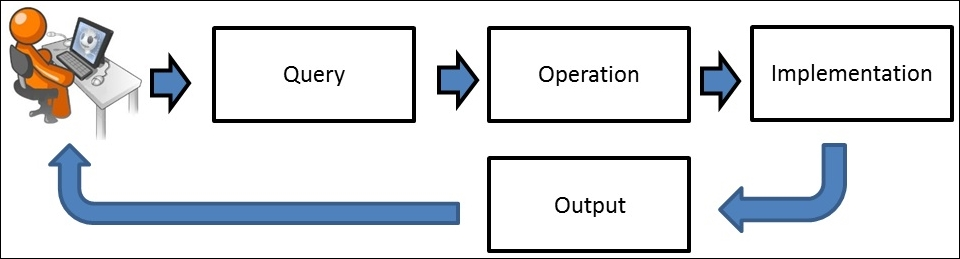Thus, ADT does not know how the data type is implemented, as these details are hidden by the user and protected from outside access, a concept referred to as encapsulation. A data structure is the implementation part of ADT, which can be implemented in any programming language. ADT can be achieved by multiple implementation strategies, as shown in Figure 1.4: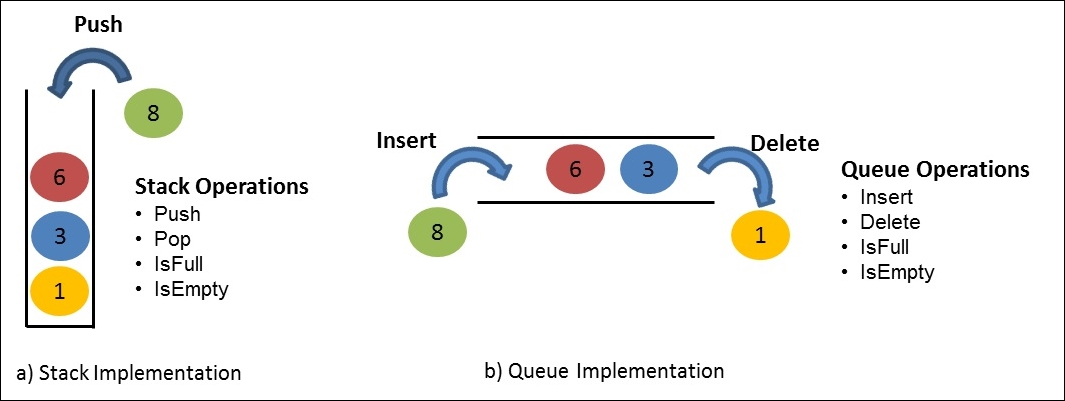Figure 1.4: Illustration of stack and queue implementation using array with integer data type

The abstraction provided by ADT helps to manage programming complexity. The ADT is also referred to as logical form, as it decides the type and operations required for implementation. The ADT is implemented by using a particular type of data structure. The data structure used to implement ADT is referred to as the physical form of data type, as it manages storage using the implementation subroutine.

## Relationship between problem and algorithm

Problem can be defined as the task to be performed. The execution of the task to be performed can be divided primarily into two components:

• Data

• Algorithm

However, there could be other controlling factors which could affect your approach development, such as problem constraints, resource constraints, computation time allowed, and so on.

The data component of the problem represents the information we are dealing with, such as numbers, text, files, and so on. For example, let's assume we want to maintain the company employee records, which include the employee name and their associated work details. This data needs to be maintained and updated regularly.

The algorithm part of a problem represents more of implementation details. It entails how we are going to manage the current data. Depending on the data and problem requirements, we select the data structure. Depending on the data structure, we have to define the algorithm to manage the dataset. For example, we can store the company employees dataset into a linked list or dictionary. Based on the defined data structure to store data approach for searching, insertion, and deletion and so on operations performed on data structure changes which is control by algorithms and implemented as program. Thus, we could state that a program is the step-by-step instructions given to a computer to do some operations:

Program = f(Algorithm, Data Structure)

To summarize, we could state that a program is the group of step-by-step instructions to solve a defined problem using the algorithm selected considering all problems and resource constraints. This book will use program representations using R to demonstrate the implementation of different data structures and algorithms.

## Basics of R

R is a statistical programming language designed and created by Ross Ihaka and Robert Gentleman. It is a derivative of the S language created at the AT&T Bell laboratories. Apart from statistical analysis, R also supports powerful visualizations. It is open source, freely distributed under a General Public License (GPL). Comprehensive R Archive Network (CRAN) is an ever-growing repository with more than 8,400 packages, which can be freely installed and used for various kinds of analysis.

R is a high-level language based on interpretations and intuitive syntax. It runs on system's or server's active memory (RAM) and stores all files, functions, and derived results in its environment as objects. The architecture utilized in R computation is shown in Figure 1.5: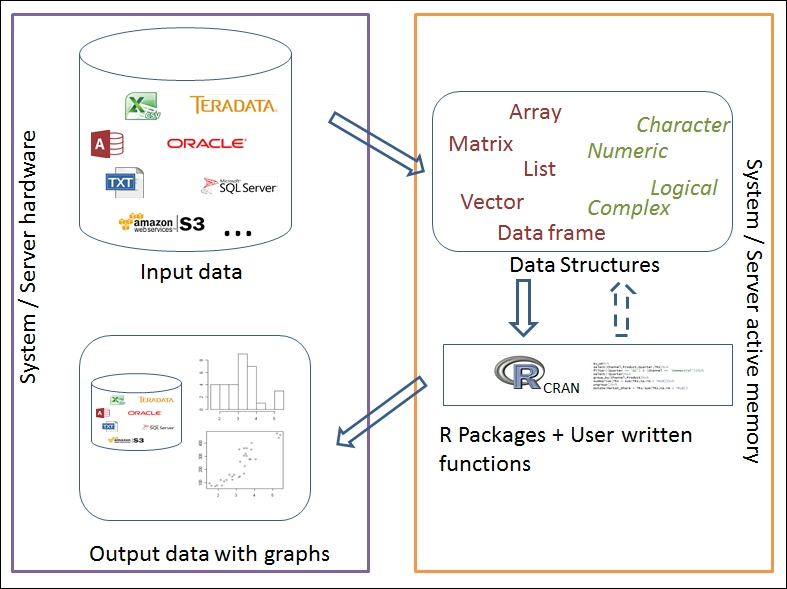Figure 1.5: Illustrate architecture of R in local/server mode

### Installation of R

R can be installed on any operating system, such as Windows, Mac OS X, and Linux. The installation files of the most recent version can be downloaded from any one of the mirror sites of CRAN: https://cran.r-project.org/. It is available under both 32-bit and 64-bit architectures.

The installation of `r-base-dev` is also highly recommended as it has many built-in functions. It also enables us to install and compile new R packages directly from the R console using the `install.packages()` command.

Upon installation, R can be called from program files, desktop shortcut, or from the command line. With default settings, the R console looks as follows: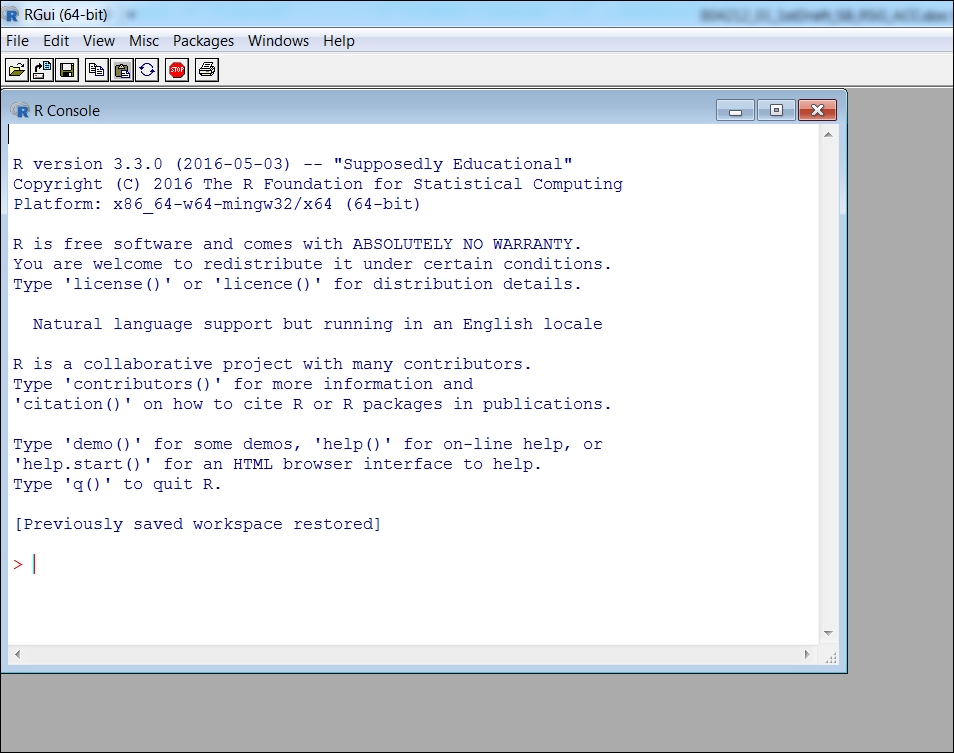Figure 1.6: The R console in which one can start coding and evaluate results instantly

R can also be used as a kernel within Jupyter Notebook. Jupyter Notebook is a web-based application that allows you to combine documentation, output, and code. Jupyter can be installed using `pip`:

```pip3 install -upgrade pip
pip3 install jupyter
```

To start Jupyter Notebook, open a shell/terminal and run this command to start the Jupyter Notebook interface in your browser:

```jupyter notebook
```

To start a new R Notebook, click on right hand side New tab and select R kernel as shown in Figure 1.7. R kernel does not come as default in Jupyter Notebook with Python as prerequisite. Anaconda distribution is recommended to install python and Jupyter and can be downloaded from https://www.continuum.io/downloads. R essentials can be installed using below command.

```conda install -c r r-essentials
```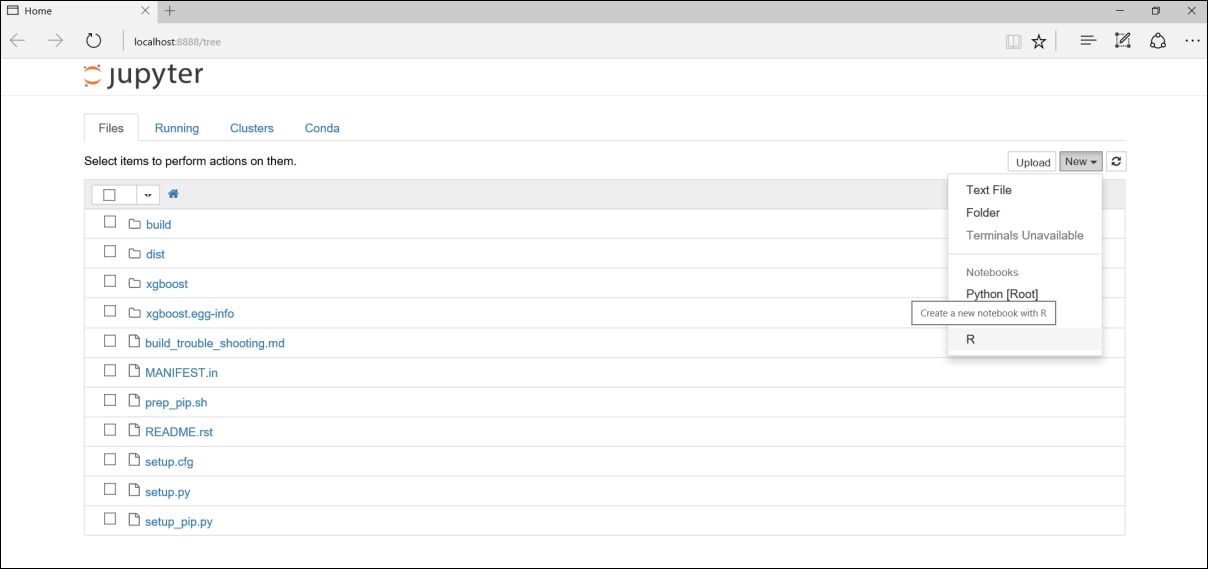Figure 1.7: Jupyter Notebook for creating R notebooks

Once notebook is opened, you could start organizing your code into cells. As R does not require formal compilation, and executes codes on runtime, one can start coding and evaluate instant results. The console view can be modified using some options under the Windows tab. However, it is highly recommended to use an Integrated Development Environment (IDE), which would provide a powerful and productive user interface for R. One such widely used IDE is RStudio, which is free and open source. It comes with its own server (RStudio Server Pro) too. The interface of RStudio is as seen in the following screenshot: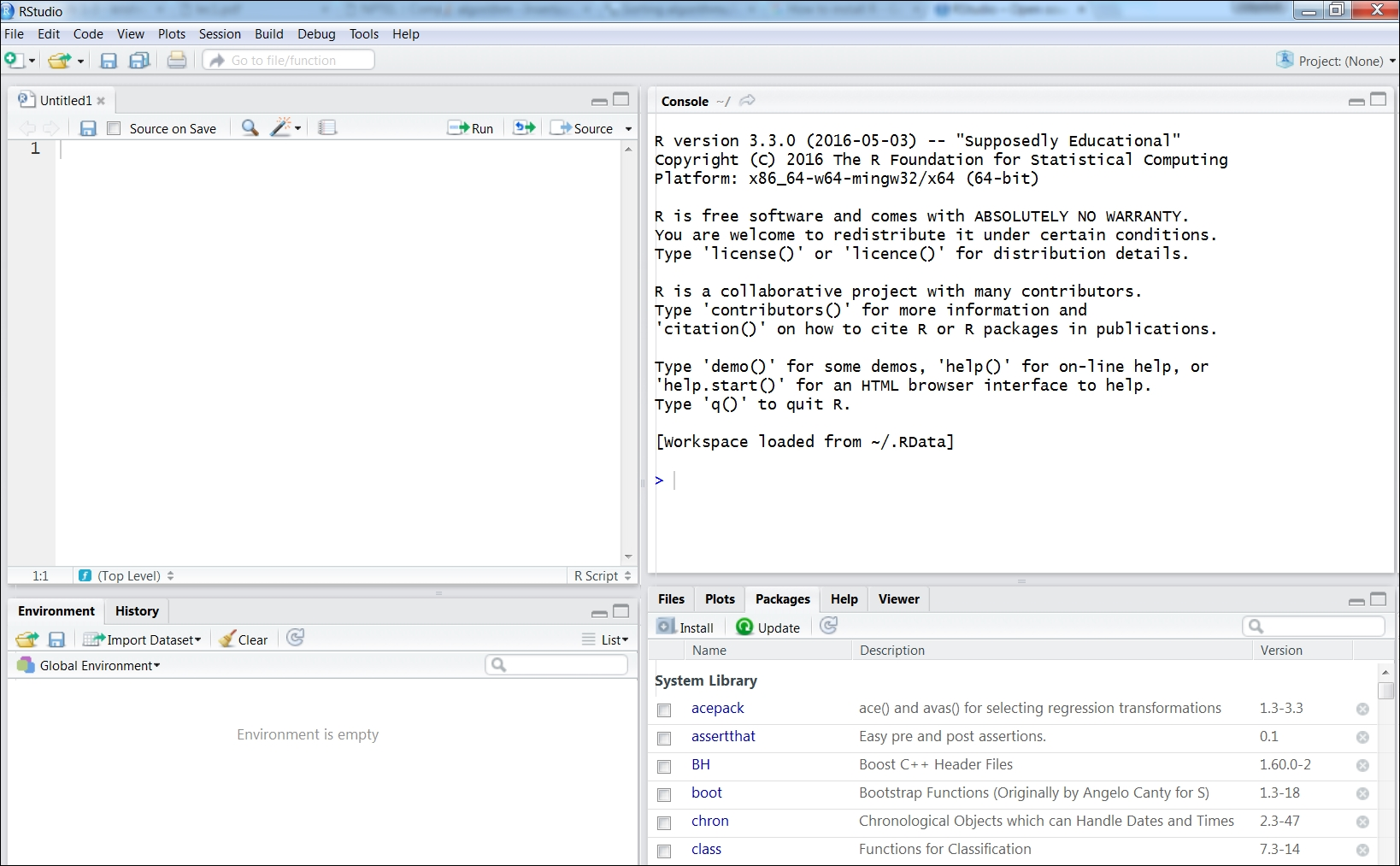Figure 1.8: RStudio, a widely used IDE for R

### Basic data types in R

R supports multiple types of data, which can be organized by dimensions and content type (homogeneous or heterogeneous), as shown in Table 1.1: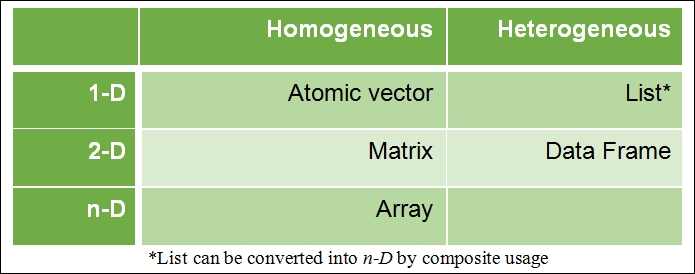Table 1.1 Basic data structure in R

Homogeneous data structure is one which consists of the same content type, whereas heterogeneous data structure supports multiple content types. All other data structures, such as data tables, can be derived using these foundational data structures. Data types and their properties will be covered in detail in Chapter 3, Linked Lists.

### Operations in R

The syntax of operators in R is very similar to other programming languages. The following is a list of operators along with their explanations.

The following table defines the various arithmetic operators: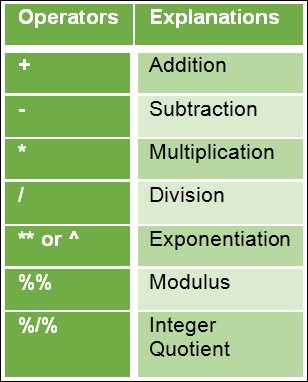Table 1.2 Basic arithmetic operators in R

The following table defines the various logical operators: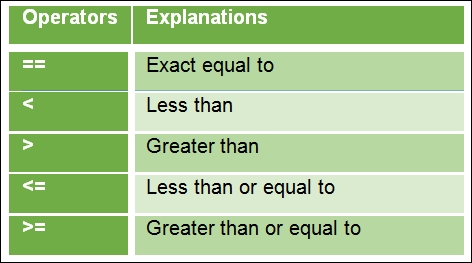Table 1.3 Basic logical operators in R

An example of assigned in R is shown below. Initially a column vector `V` is assigned followed by operations on column vector such as addition, subtraction, square root and log. Any operation applied on column vector is element-wise operation.

```> V <- c(1,2,3,4,5,6)  ## Assigning a vector V
> V
 1 2 3 4 5 6
> V+10 ## Adding each element in vector V with 10
 11 12 13 14 15 16
> V-1  ## Subtracting each element in vector V with 1
 0 1 2 3 4 5
> sqrt(V)  ## Performing square root operation on each element in  vector V
 1.000000 1.414214 1.732051 2.000000 2.236068 2.449490
> V1 <- log(V)  ## Storing log transformed of vector V as V1
> V1
 0.0000000 0.6931472 1.0986123 1.3862944 1.6094379 1.7917595
```

### Control structures in R

Control structures such as loops and conditions form an integral part of any programming language, and R supports them with much intuitive syntax.

#### If condition

The syntax for the `if` condition is as follows:

```    if (test expression)
{
Statement upon condition is true
}
```

If the test expression is `true`, the statement is executed. If the statement is `false`, nothing happens.

In the following example, an atomic vector `x` is defined, and it is subjected to two conditions separately, that is, whether `x` is greater than `5` or less than `5`. If either of the conditions gets satisfied, the value `x` gets printed in the console following the corresponding `if` statement:

```> x <- 10
> if (x < 5) print(x)
> if (x > 5) print(x)
 10
```

#### If...else condition

The syntax for the `if...else` condition is as follows:

```    if(test expression)
{
Statement upon condition is true
} else {
Statement upon condition is false
}
```

In this scenario, if the condition in the test expression is `true`, the statement under the `if` condition is executed, otherwise the statement under the `else` condition is executed.

The following is an example in which we define an atomic vector `x` with a value `10`. Then, we verify whether `x,` when divided by `2,` returns `1` (R reads `1` as a Boolean True). If it is True, the value `x` gets printed as an odd number, else as an even number:

```> x=10
> if (x %% 2)
+ {
+   print(paste0(x, " :odd number"))
+ } else {
+   print(paste0(x, " :even number"))
+ }
 "10 :even number"
```

#### Ifelse function

An `ifelse()` function is a condition used primarily for vectors, and it is an equivalent form of the `if...else` statement. Here, the conditional function is applied to each element in the vector individually. Hence, the input to this function is a vector, and the output obtained is also a vector. The following is the syntax of the `ifelse` condition:

```    ifelse (test expression, statement upon condition is true,
statement upon condition is false)```

In this function, the primary input is a test expression which must provide a logical output. If the condition is `true`, the subsequent statement is executed, else the last statement is executed.

The following is example in which a vector `x` is defined with integer values from 1 to 7. Then, a condition is applied on each element in vector `x` to determine whether the element is an odd number or an even number:

```> x <- 1:6
> ifelse(x %% 2, paste0(x, " :odd number"), paste0(x, " :even  number"))
 "1 :odd number"  "2 :even number" "3 :odd number"
 "4 :even number" "5 :odd number"  "6 :even number"
```

#### For() loop

A `for` loop is primarily used to iterate a statement over a vector of values in a given sequence. The vector can be of any type, that is, numeric, character, Boolean, or complex. For every iteration, the statement is executed.

The following is the syntax of the `for` loop:

```    for(x in sequence vector)
{
Statement to be iterated
}
```

The loop continues till all the elements in the vector get exhausted as per the given sequence.

The following example details a `for` loop. Here, we assign a vector `x,` and each element in vector `x` is printed in the console using a `for` loop:

```> x <- c("John", "Mary", "Paul", "Victoria")
> for (i in seq(x)) {
+   print(x[i])
+ }
 "John"
 "Mary"
 "Paul"
 "Victoria"
```

#### Nested for( ) loop

The nested `for` loop can be defined as a set of multiple `for` loops within each `for` loop as shown here:

```    for( x in sequence vector)
{
First statement to be iterated
for(y in sequence vector)
{
Second statement to be iterated
.........
}
}
```

In a nested `for` loop, each subsequent `for` loop is iterated for all the possible times based on the sequence vector in the previous `for` loop. This can be explained using the following example, in which we define mat as a matrix (3x3), and our desired objective is to obtain a series of summations which will end up with the total sum of all the values within the matrix. Firstly, we initialize `sum` to `0`, and then subsequently, `sum` gets updated by adding itself to all the elements in the matrix in a sequence. The sequence is defined by the nested `for` loop, wherein for each row in a matrix, each of the values in all the columns gets added to sum:

```> mat <- matrix(1:9, ncol = 3)
> sum <- 0
> for (i in seq(nrow(mat)))
+ {
+   for (j in seq(ncol(mat)))
+   {
+     sum <- sum + mat[i, j]
+     print(sum)
+   }
+ }
 1
 5
 12
 14
 19
 27
 30
 36
 45
```

#### While loop

In R, `while` loops are iterative loops with a specific condition which needs to be satisfied. The syntax of the `while` loop is as follows:

```    while (test expression)
{
Statement upon condition is true (iteratively)
}
```

Let's understand the `while` loop in detail with an example. Here, an object `i` is initialized to `1`. The test expression which needs to be satisfied for every iteration is `i<10`. Since `i` = `1`, the condition is TRUE, and the statement within the `while` loop is evaluated. According to the statement, `i` is printed on the console, and then increased by `1` unit. Now `i` increments to `2,` and once again, the test expression, whether the condition (`i < 10`) is true or false, is checked. If TRUE, the statement is again evaluated. The loop continues till the condition becomes false, which, in our case, will happen when `i` increments to `10`. Here, incrementing `i` becomes very critical, without which the loop can turn into infinite iterations:

```> i <- 1
> while (i < 10)
+ {
+   print(i)
+   i <- i + 1
+ }
 1
 2
 3
 4
 5
 6
 7
 8
 9
```

#### Special statements in loops

In R, the loops can be altered using `break` or `next` statements. This helps in inducing other conditions required within the statement inside the loop.

##### Break statement

The syntax for the Break statement is `break`. It is used to terminate a loop and stop the remaining iterations. If a `break` statement is provided within a nested loop, then the innermost loop within which the `break` statement is mentioned gets terminated, and iterations of the outer loops are not affected.

The following is an example in which a `for` loop is terminated when i reaches the value `8`:

```> for (i in 1:30)
+ {
+   if (i < 8)
+   {
+     print(paste0("Current value is ",i))
+   } else {
+     print(paste0("Current value is ",i," and the loop breaks"))
+     break
+   }
+ }
 "Current value is 1"
 "Current value is 2"
 "Current value is 3"
 "Current value is 4"
 "Current value is 5"
 "Current value is 6"
 "Current value is 7"
 "Current value is 8 and the loop breaks"
```
##### Next statement

The syntax for a Next statement is `next`. The `next` statements are used to skip intermediate iterations within a loop based on a condition. Once the condition for the `next` statement is met, all the subsequent operations within the loop get terminated, and the next iteration begins.

This can be further explained using an example in which we print only odd numbers based on a condition that the printed number, when divided by `2`, leaves the remainder `1`.

```> for (i in 1:10)
+ {
+   if (i %% 2)
+   {
+     print(paste0(i, " is an odd number."))
+   } else {
+     next
+   }
+ }
 "1 is an odd number."
 "3 is an odd number."
 "5 is an odd number."
 "7 is an odd number."
 "9 is an odd number."
```

#### Repeat loop

The `repeat` loop is an infinite loop which iterates multiple times without any inherent condition. Hence, it becomes mandatory for the user to explicitly mention the terminating condition, and use a `break` statement to terminate the loop.

The following is the syntax for a `repeat` statement:

```    repeat
{
Statement to iterate along with explicit terminate condition
including break statement
}
```

In the current example, `i` is initialized to `1`. Then, a `for` loop iterates, within which an object cube is evaluated and verified using a condition whether the cube is greater than `729` or not. Simultaneously, `i` is incremented by `1` unit. Once the condition is met, the `for` loop is terminated using a `break` statement:

```> i <- 1
> repeat
+ {
+   cube <- i ** 3
+   i <- i + 1
+   if (cube < 729)
+   {
+     print(paste0(cube, " is less than 729. Let's remain in the
loop."))
+   } else {
+     print(paste0(cube, " is greater than or equal to 729. Let's exit
the loop."))
+     break
+   }
+ }
 "1 is less than 729. Let's remain in the loop."
 "8 is less than 729. Let's remain in the loop."
 "27 is less than 729. Let's remain in the loop."
 "64 is less than 729. Let's remain in the loop."
 "125 is less than 729. Let's remain in the loop."
 "216 is less than 729. Let's remain in the loop."
 "343 is less than 729. Let's remain in the loop."
 "512 is less than 729. Let's remain in the loop."
 "729 is greater than 729. Let's exit the loop."
```

## First class functions in R

R is primarily a functional language at its core. In R, functions are treated just like any other data types, and are considered as first-class citizens. The following example shows that R considers everything as a function call.

Here, the operator `+` is a function in itself:

```> 10+20
 30
> "+"(10,20)
 30
```

Here, the operator `^` is also a function in itself:

```> 4^2
 16
> "^"(4,2)
 16

```

Now, let's dive deep into functional concepts, which are crucial and widely used by R programmers.

Vectorized functions are among the most popular functional concepts which enable the programmer to execute functions at an individual element level for a given vector. This vector can also be a part of dataframe, matrix, or a list. Let's understand this in detail using the following example, in which we would like to have an operation on each element in a given vector `V_in`. The operation is to square each element within the vector and output it as vector `V_out`. We will implement them using three approaches as follows:

Approach 1: Here, the operations will be performed at the element level using a `for` loop. This is the most primitive of all the three approaches in which vector allocation is being performed using the style of S language:

```> V_in <- 1:100000         ## Input Vector
> V_out <- c()             ## Output Vector
> for(i in V_in)           ## For loop on Input vector
+ {
+   V_out <- c(V_out,i^2)  ## Storing on Output vector
+ }
```

Approach 2: Here, the vectorized functional concept will be used to obtain the same objective. The loops in vectorized programming are implemented in C language, and hence, perform much faster than `for` loops implemented in R (Approach 1). The time elapsed to run this operation is instantaneous:

```> V_in <- 1:100000    ## Input Vector
> V_out <- V_in^2     ## Output Vector
```

Approach 3: Here, higher order functions (or nested functions) are used to obtain the same objective. As functions are considered first class citizens in R, these can be called as an argument within another function. The widely used nested functions are in the apply family. The following table provides a summary of the various types of functions within the apply family: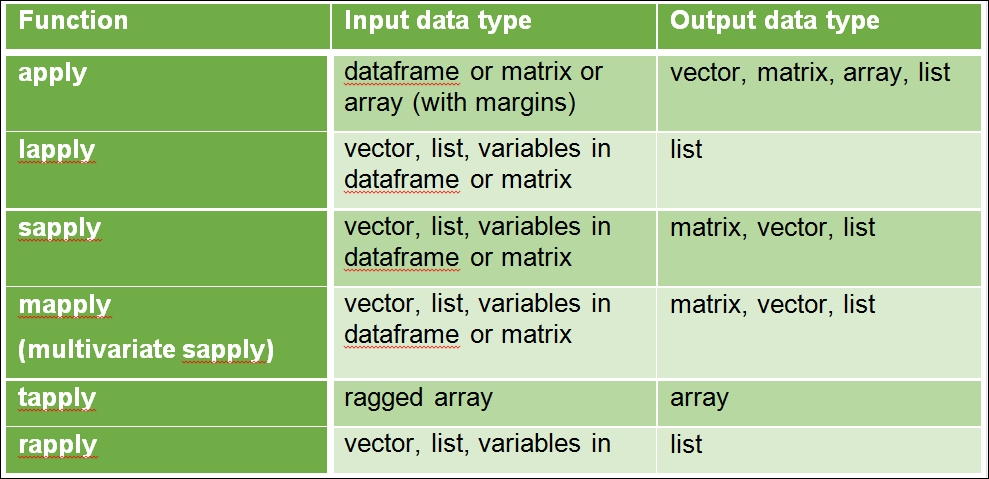Table 1.4 Various types of functions in the apply family

Now, lets' evaluate the first class function through examples. An `apply` function can be applied to a dataframe, matrix, or array. Let's illustrate it using a matrix:

```> x <- cbind(x1 = 7, x2 = c(7:1, 2:5))
> col.sums <- apply(x, 2, sum)
> row.sums <- apply(x, 1, sum)
```

The `lapply` is a first class function to be applied to a vector, list, or variables in a dataframe or matrix. An example of `lapply` is shown below:

```> x <- list(x1 = 7:1, x2 = c(7:1, 2:5))
> lapply(x, mean)
```

The use of the `sapply` function for a vector input using customized function is shown below:

```> V_in <- 1:100000 ## Input Vector
> V_out <- sapply(V_in,function(x) x^2) ## Output Vector
```

The function `mapply` is a multivariate `sapply`. The `mapply` function is the first input, followed by input parameters as shown below:

```mapply(FUN, ..., MoreArgs = NULL, SIMPLIFY = T, USE.NAMES = T)
```

An example of `mapply` to replicated two vector can be obtained as:

```> mapply(rep, 1:6, 6:1)
```

The function call `rep` function in R with input from 1 to 6 and is replicated as 6 to 1 using the second dimension of the `mapply` function. The `tapply` applies a function to each cell of the ragged array. For example, let's create a list with a multiple array: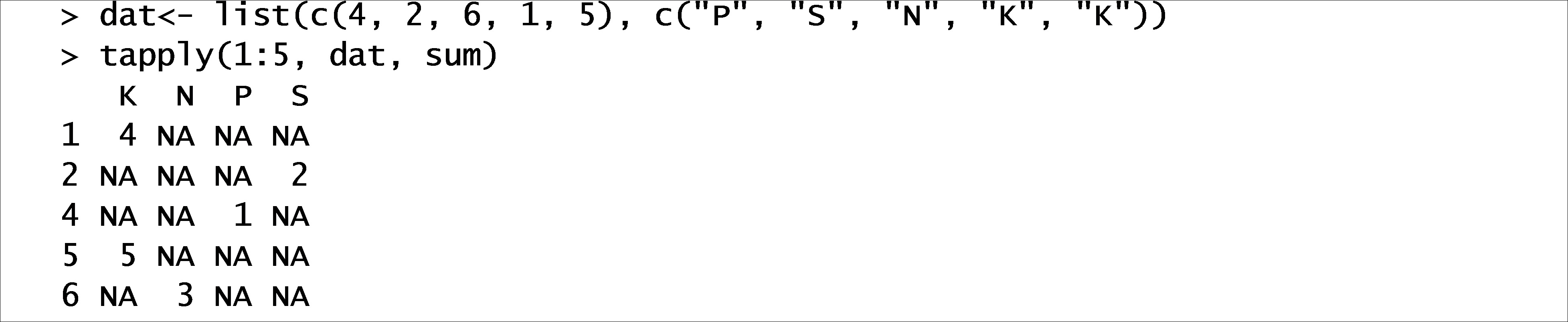The output is a relationship between two vectors with position as a value. The function `rapply` is a recursive function for lapply as shown below:

```> X <- list(list(a = pi, b = list(c = 1:1)), d = "a test")
> rapply(X, sqrt, classes = "numeric", how = "replace")
```

The function applies sqrt to all numeric classes in the list and replace it with new values.

## Exercises

1. Can you think of ways in which we can extract a few attributes (columns) and observations (rows) based on a certain condition?

2. Can we add multiple arguments within a function in the `apply` family? If yes, what is the syntax for assigning multiple arguments?

3. A general notion states that `for` loops are slower than the `apply` functions. Is it true or false? If false, what are the conditions in which the notion gets negated?

4. Define ADT for calculating the area of any geometrical object, such as a circle, square, and so on.

## Summary

Computational power has been continuously increasing in the last couple of decades, and so does the amount of data captured by different industries. To cope with data size, faster and efficient information retrieval is an eminent requirement.

In this chapter, you were introduced to ADT and data structure. ADT is used to define high-level features and operations representing different data structures, and algorithms are used to implement ADT. A data type should be atomic, traceable, accurate, and have clear and concise characteristic properties for efficiency along with unambiguity. You also learned the basics of R, including data type, conditional loops, control structure, and first class functions.

The computational time taken by an algorithm is most important objective considered while selecting data structures and algorithms. The next chapter will provide the fundamentals for the analysis of algorithms.

• ##### Dr. PKS Prakash

Dr. PKS Prakash is a data scientist and author.

He has spent the last 12 years in developing many data science solutions in several practical areas in healthcare, manufacturing, pharmaceuticals, and e-commerce. He currently works as the data science manager at ZS Associates. He is the co-founder of Warwick Analytics, a spin-off from University of Warwick, UK. Prakash has published articles widely in research areas of operational research and management, soft computing tools, and advanced algorithms in leading journals such as IEEE-Trans, EJOR, and IJPR, among others. He has edited an article on Intelligent Approaches to Complex Systems and contributed to books such as Evolutionary Computing in Advanced Manufacturing published by WILEY and Algorithms and Data Structures using R and R Deep Learning Cookbook, published by PACKT.

Browse publications by this author
• ##### Achyutuni Sri Krishna Rao

Achyutuni Sri Krishna Rao is a Data Scientist, a Civil Engineer and an Author. He has spent last 4 years in developing many data science solutions to solve problems from leading companies in healthcare, pharmaceutical and manufacturing domain. He is working as Data Science Consultant at ZS Associates.

Sri Krishna’s background involves a masters in Enterprise Business Analytics and Machine Learning from National University of Singapore, Singapore. His other educational background involves a Bachelor’s from National Institute of Technology Warangal, India.

Sri Krishna has published widely in research areas of civil engineering. He has contributed in a book titled “Algorithms and Data Structures using R” published by PACKT.

Browse publications by this author